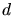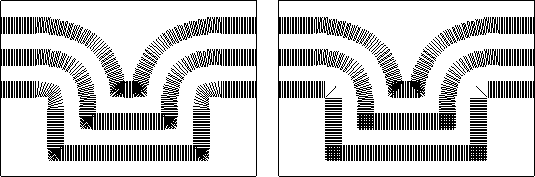Prev: 2.3.1 Spherical Segment Elements Up: 2.3 Optimizations for the Next: 2.3.3 Benchmark Example and

## 2.3.2 Line Elements

A further possibility for CPU time reduction is the restriction to exclusive application of lines as structuring elements. The length of the lines is determined by the deposition rate multiplied with the time-step. In case of isotropic deposition the growth direction is perpendicular to the surface, therefore the surface orientation which is not implicitly given in the cellular material representation has to be calculated. The calculation of the orientation of a surface cell is done by averaging the normals of the cube faces of all surface cells within a certain distancefrom the considered surface cell. Averaging alone would give wrong results where planar faces intersect, since the surface normals of surface cells located within averaging distancefrom an intersection would already start to incline towards the normal of the second plane. This can be seen at the convex corner of the initial (lowest) geometry and at the concave corners on the left hand side of Fig. 2.5, which depicts the unit surface normal vectors for the surface cells of a cylindrical via for two time-steps of an isotropic deposition. By means of a special edge detection algorithm it is possible to conserve sharp corners of the geometry, which otherwise would be rounded by the averaging algorithm. The right hand side in Fig. 2.5 shows the surface normals corrected in this way for the same cylindric via structure.The line element method is similar to ray-tracing topography algorithms. If the lines corresponding to the rays are dense enough with respect to the cellular geometry representation, a smooth surface is assured. Special care has to be taken at corners or edges where the line elements have to be applied repeatedly with directions interpolated between the surface orientation of the adjacent cells for assuring a smooth and continuous surface. The interpolation at edges is completely different from the averaging method used for the calculation of the surface normals (see left hand side of Fig. 2.5). Using the uncorrected, averaged surface normals would not correctly reproduce the isotropically deposited layer.

In contrast to the spherical segment algorithm introduced before, this algorithm performs better for curved surfaces. Only cells very close to the surface are hit repeatedly when interpolation is necessary at curved regions. The right hand side of Fig. 2.4 where the linear structuring elements are applied at the same positions as the spherical segments on the left hand side, gives an idea of the further reduction of redundant operations achievable with this algorithm.

W. Pyka: Feature Scale Modeling for Etching and Deposition Processes in Semiconductor Manufacturing